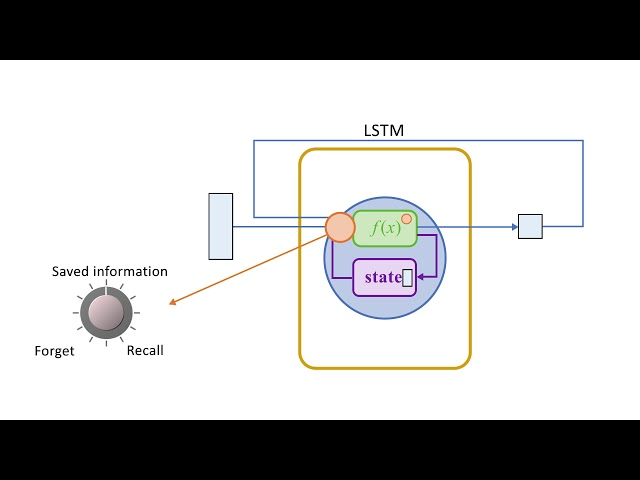# What is Convolutional Long Short-Term Memory (ConvLSTM)

##### Understanding Convolutional Long Short-Term Memory (ConvLSTM)

The Convolutional Long Short-Term Memory (ConvLSTM) is a type of neural network that combines the Convolutional Neural Network (CNN) and the Long Short-Term Memory (LSTM) model. CNNs are typically used for image processing tasks whereby they identify and extract features from images. LSTM models, on the other hand, are used for time-series analysis, where they utilize memory cells to retain information over time. The ConvLSTM is designed to combine these two models to handle spatiotemporal data that involve both spatial and temporal dependencies.

The ConvLSTM was first introduced by Xingjian Shi et al. in a 2015 paper titled "Convolutional LSTM Network: A Machine Learning Approach for Precipitation Nowcasting." The paper proposed the use of the ConvLSTM model for precipitation forecasting using radar images, which demonstrated that the model outperformed traditional LSTM models in terms of accuracy and handling spatiotemporal data.

##### How ConvLSTM Works

The ConvLSTM architecture works by maintaining the spatial information of its input while also learning temporal dependencies. Typically, CNNs lose spatial information as they process the input images due to convolution and pooling layers. LSTM models, on the other hand, need to maintain temporal information, meaning they require the input sequences to be ordered. The ConvLSTM model uses a combination of convolutional and LSTM layers to retain both spatial and temporal information.

The architecture of the ConvLSTM model consists of four main components:

• Convolutional Layers
• Forget Gate
• Input Gate
• Output Gate
##### Convolutional Layers

The Convolutional layers in the model perform the same role as in a standard CNN by extracting relevant features from the input image. The convolutional layers maintain the spatial information of the input image throughout the model, unlike a standard LSTM model. Convolutional layers used in the ConvLSTM model are typically the same as those used in a standard CNN model.

##### Forget Gate

The Forget Gate is responsible for deciding what information to forget from the memory cell of the LSTM model. It takes in the input from the previous time step and the current pixel value and produces an output between 0 and 1. A value of 0 represents that the model should forget the previous information, while a value of 1 indicates that the information should be retained. The forget gate takes into account the input and the hidden state from the previous time step.

##### Input Gate

The Input Gate is responsible for deciding what new information to add to the memory cell. It takes in the input from the previous time step and the current pixel value and produces an output between 0 and 1. A value of 0 indicates that no new information should be added, while a value of 1 represents that all new information should be retained.

##### Output Gate

The Output Gate is responsible for outputting the hidden state at the current time step. It takes in the input from the previous time step and the current pixel value, together with the memory-cell state, and produces an output between 0 and 1. The output is then multiplied by the hyperbolic tangent of the memory cell to obtain the new hidden state. The output gate helps to determine the amount of information to pass to the next time step.

##### Applications of ConvLSTM

The ConvLSTM model has been applied in several domains, primarily those involving spatiotemporal data. A few examples of these applications are highlighted below:

• Weather Forecasting: ConvLSTM has been used extensively in weather forecasting to accurately predict weather patterns, such as rainfall and temperature.
• Traffic Prediction: Traffic flow prediction is a popular application of ConvLSTM. The model utilizes historical data to make future predictions about traffic conditions.
• Bioinformatics: ConvLSTM has been used to predict the behavior of biological molecules, such as protein folding and DNA sequencing.
• Video Processing: The ConvLSTM model has been applied in video processing, where it analyzes and processes video data to identify patterns for subsequent action.
##### Conclusion

The ConvLSTM model is a powerful neural network architecture that combines the convolutional and LSTM models to handle spatiotemporal data. By retaining both spatial and temporal information, the model is able to make accurate predictions in domains such as traffic prediction, bioinformatics, weather forecasting, and video processing. The model has been applied in several real-world scenarios, proving its versatility and effectiveness.